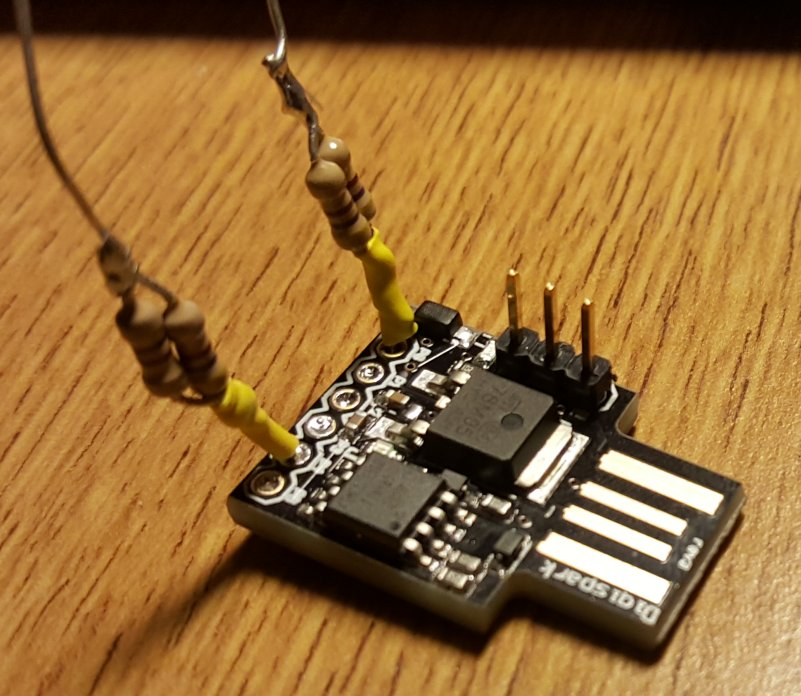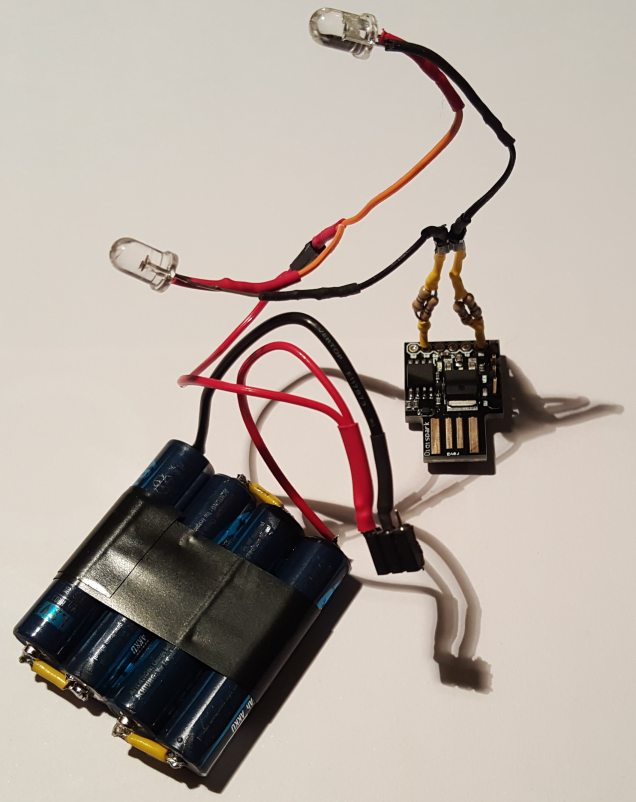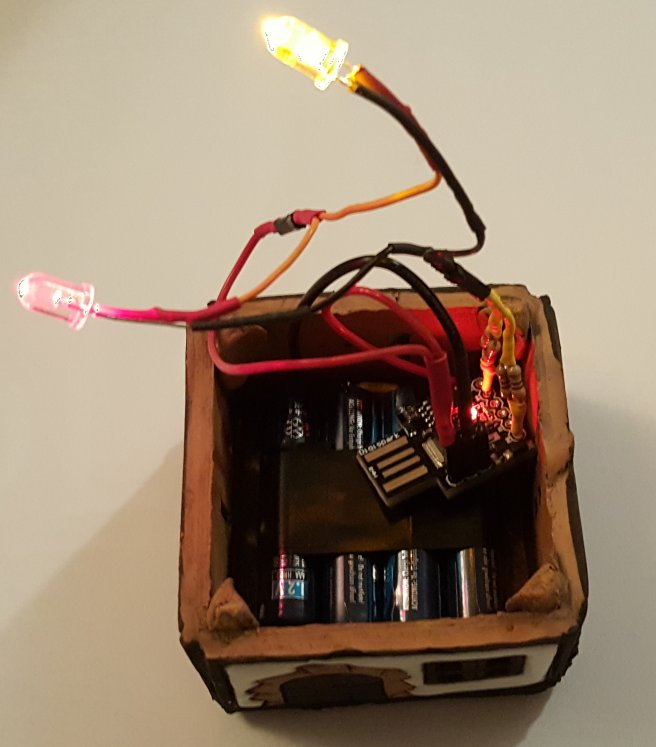# Difference between revisions of "Realistic Fire Simulation"

In this page is described how to create a realistic flickering fire effect with LEDs and an Arduino board. In this case I used a Digispark due to its small size.

The intention is to give some light to a small clay decoration house, one of those prepared for lighting a small candle inside and evaporate water with essence. Instead of using a candle I put a simple battery with some LEDs inside but it looked too boring so I tried to imitate the movement of a candle inside it. Here it is described the design and process followed.

## Component Schematics

The three LEDs (two reds and one yellow - I guess one red and two yellows would also look nice) are connected to the PWM ports of the arduino (Digispark, which has exactly 3 PwM pins).

The pin P1 of the Digispark board already has red LED attached to it, so there is no need to add a new one, or to remove the current one. For this reason the new schematics is:

## Building

In the next picture the connections for the LEDs in pins 0 and 4 have been soldered. Each pin has two 470 ohm resistors in parallel that make a total of 235 ohm (I did not have 220 ohm or similar). As the system is powered by 4.8v that makes a maximum of a bit more of 20 mA per LED, which should be bright enough and safe for the microcontroller. Visit the next section to have a look at code running in the board.The next picture shows the system already assembled, the two conventional LEDs plus the one included in the board, with a 4x AAA 1.2v battery pack (giving a total of 4.8v). The battery pack has to be connected to the 5v and GND connector of the Digispark board (4.8v is enough). The Digispark board has a power on red LED that has been removed with a sharp knife because the continuous red light was spoiling the fire effect.This picture shows the powered on system inside the bottom of the clay house, with the three lights already flickering.## Code

For schematic 1, with all LEDs logically inverted:

```int p1 = 0; // Set the 3 pins with PWM
int p2 = 1;
int p3 = 4;

void setup() {
pinMode(p1, OUTPUT); // Set the pins as output
pinMode(p2, OUTPUT);
pinMode(p3, OUTPUT);
}

void loop() {
int v1, v2, v3;

v1 = random(155) + 100; // Calculate the new light
v2 = random(155) + 100; // intensity values for each LED
v3 = random(155) + 100;

analogWrite(p1, 255 - v1); // Apply the light intensity
analogWrite(p2, 255 - v2); // to each LED
analogWrite(p3, 255 - v3);

delay(20 + random(80)); // Wait some random time for next udpate
}
```

For schematic 2 (reusing the LED attached to Pin 1, which is not "logically inverted"):

```int p1 = 0; // Set the 3 pins with PWM
int p2 = 1;
int p3 = 4;

void setup() {
pinMode(p1, OUTPUT); // Set the pins as output
pinMode(p2, OUTPUT);
pinMode(p3, OUTPUT);
}

void loop() {
int v1, v2, v3;

v1 = random(155) + 100; // Calculate the new light
v2 = random(155) + 100; // intensity values for each LED
v3 = random(155) + 100;

analogWrite(p1, 255 - v1); // Apply the light intensity to each  LED
analogWrite(p2, v2);       // This LED is not "logically inverted"
analogWrite(p3, 255 - v3);

delay(20 + random(80)); // Wait some random time for next udpate
}
```